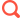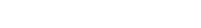# 帝国cms高级应用，对系统设置的重新赋值妙用

上一篇我们学习了帝国cms变量赋值的技巧，这里我们再来看一下对系统设置的重新赋值，这样做的目的只有一个，就是不修改系统文件，使二次开发更为绿色，不影响后续的系统升级。

## 一、帝国cms的全局变量

我们在模板中放入代码<?=\$GLOBALS?>，比如网站首页模板中，刷新相应的页面，右键查看网页源代码，就可以在源码中看到帝国cms的全局变量和数组，这其中有 \$public_r(系统设置数组)，\$class_r(栏目设置数组)，\$emod_r(模型设置数组)，\$fun_r(函数语言数组)，\$level_r(会员组数组)等等，上一篇文章中我们就是对\$fun_r中的一个成员重新赋值实现了不修改源文件的英文列表分页效果。

数组的概念：

数组就是一组数据的集合，把一系列数据组织起来，形成一个可操作的整体。数组的每个实体都包含两项：键和值。

这里举一个生动的例子，周星驰的《唐伯虎点秋香》电影中，唐伯虎混入华府做了低等下人，当时武状元说“小子，从今天开始你就是华府的低等下人，9527就是你的终身代号。”这里的低等下人就是一个数组，他指的是一个整体而非一个，其中9527就是数组一个成员的KEY值，唐伯虎这个人就是9527这个KEY的VALUE值；我们把低等下人这个数组写成PHP数组格式，请看下面的代码：上面明白了什么是数字，下面我们再来看一下帝国cms的数组，找到/e/class/config.php文件并打开，在其中搜索\$public_r，这里的\$pulic_r就是一个数组，存储了帝国cms的系统设置，下图是部分\$public_r数字键值的截图，sitename,newsurl都是key，对应的帝国网站管理系统是这个键（key）的VALUE值；你可以在任意模板中加入<?=\$public_r[KEY值]?>重新生成页面可以得到相对应的VALUE值，比如<?=\$public_r[newsurl]?>得到你的网站地址；## 对系统设置进行重新赋值的用途

1、这个列表页用的分页标签是[!–show.listpage–]，为帝国cms的默认分页变量

2、对应的这个分页变量在\$public_r数组中键名为listpagelistfun

3、在/e/class/userfun.php写入一个新的分页函数，cmsdx_listpage()，完整代码如下（由系统自带的列表式分页函数修改而来）：

1. <?php
2. //test listpage
4.     global \$fun_r,\$public_r;
5.     if(\$num<=\$line)
6.     {
7.         \$pager['showpage']='';
8.         return \$pager;
9.     }
10.     \$page_line=\$public_r['listpagelistnum'];
11.     \$snum=2;
12.     //\$totalpage=ceil(\$num/\$line);//取得总页数
13.     \$firststr='<a title="Total record"> <b>'.num2str(\$num).'</b> </a>  ';
14.     //上一页
15.     if(\$page<>1)
16.     {
18.         \$pagepr=\$page-1;
19.         if(\$pagepr==1)
20.         {
21.             \$prido="index".\$type;
22.         }
23.         else
24.         {
25.             \$prido="index_".\$pagepr.\$type;
26.         }
28.     }
29.     //下一页
30.     if(\$page!=\$totalpage)
31.     {
32.         \$pagenex=\$page+1;
35.     }
36.     \$starti=\$page-\$snum<1?1:\$page-\$snum;
37.     \$no=0;
38.     for(\$i=\$starti;\$i<=\$totalpage&&\$no<\$page_line;\$i++)
39.     {
40.         \$no++;
41.         if(\$page==\$i)
42.         {
43.             \$is_1="<b>";
44.             \$is_2="</b>";
45.         }
46.         elseif(\$i==1)
47.         {
49.             \$is_2="</a>";
50.         }
51.         else
52.         {
54.             \$is_2="</a>";
55.         }
56.         \$returnstr.=' '.\$is_1.num2str(\$i).\$is_2;
57.     }
58.     \$returnstr=\$firststr.\$toppage.\$prepage.\$returnstr.\$nextpage.\$lastpage;
59.     \$pager['showpage']=\$returnstr;
60.     return \$pager;
61. }
62.
63.
64. function num2str(\$num) {
65.     if(!is_numeric(\$num)) return false;
66.
67.     \$ret = '';
68.     if(\$num < 0) {
69.         \$ret = '负';
70.         \$num = -\$num;
71.     }
72.
73.     \$zhNumArray = array(
74.         "1" => "一",
75.         "2" => "二",
76.         "3" => "三",
77.         "4" => "四",
78.         "5" => "五",
79.         "6" => "六",
80.         "7" => "七",
81.         "8" => "八",
82.         "9" => "九",
83.         "0" => "零",
84.     );
85.
86.     \$bitStrArray = array(
87.         "1" => "",
88.         "10" => "十",
89.         "100" => "百",
90.         "1000" => "千",
91.         "10000" => "万",
92.         "100000000" => "亿",
93.     );
94.
95.     \$prebit = 0;//上次记录的位数
96.     krsort(\$bitStrArray);
97.     foreach(\$bitStrArray as \$bit => \$name) {
98.         \$bit = floatval(\$bit);
99.         if(\$num >= \$bit) {
100.             \$tmpNum = floor(\$num / \$bit);
101.             if(\$tmpNum >= 10) {
102.                 \$tmpRet = num2str(\$tmpNum);
103.                 \$ret .= \$tmpRet . \$name;
104.             } else {
105.                 if(\$prebit/\$bit > 10) {//按照中文习惯补零
106.                     \$ret .= '0';
107.                 }
108.                 \$ret .= \$tmpNum . \$name;
109.             }
110.             \$prebit = \$bit;
111.             \$num = fmod(\$num\$bit);//取模
112.         }
113.     }
114.
115.     \$ret = str_replace(array_keys(\$zhNumArray), array_values(\$zhNumArray), \$ret);
116.     return \$ret;
117. }
118. ?>

4、接下来我们要在相应的列表页模板最顶部加入赋值代码<?php \$public_r[listpagelistfun]=”cmsdx_listpage”;?>(备注：这里一定要使用双引号，否则会提示函数未定义)

(说明：网站后台 - 系统参数设置 - 信息设置 - 列表分页函数(列表) 默认填写的函数是sys_ShowListMorePage，我们现在需要在某个栏目列表页使用另外的函数，而大部分栏目页仍然采用默认的函数，所以我们在userfun.php文件中封装了一个新的函数，并且将函数名赋值给了\$public_r[listpagelistfun]，这样使用这个列表页模板的栏目都会使用这个新的分页函数了）

## 总结

Tags： 系统设置   赋值

### 相关推荐